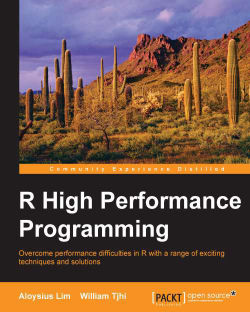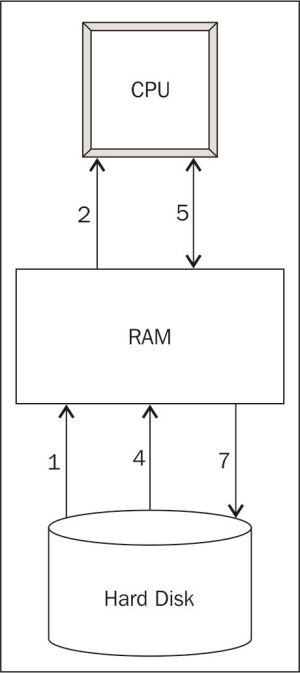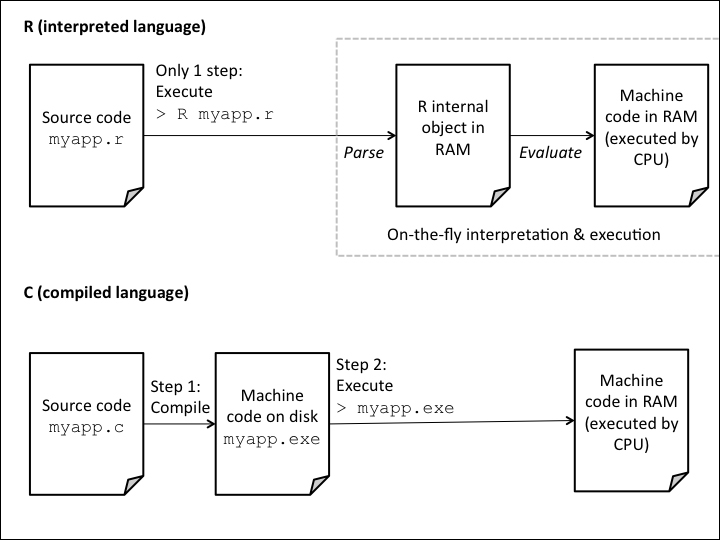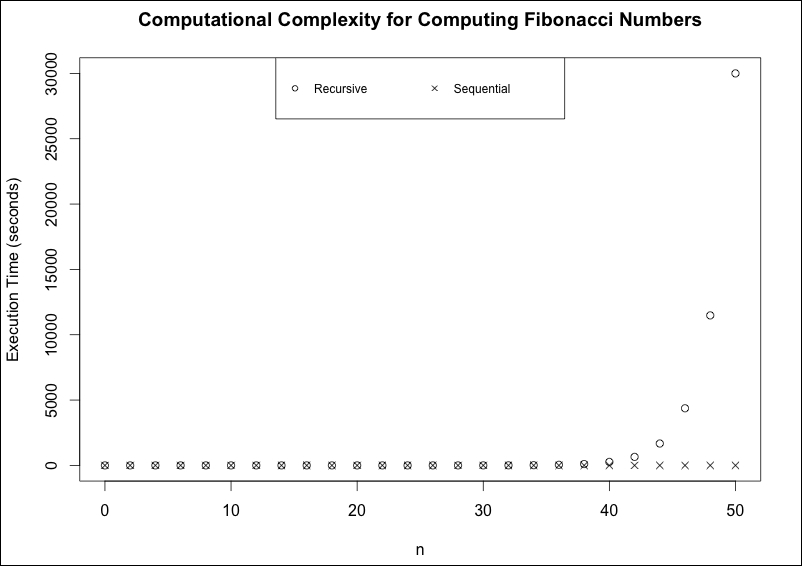### R High Performance Programming4 (1 reviews total)
By Aloysius Lim , William Tjhi

• Constantly updated with 100+ new titles each month
• Breadth and depth in over 1,000+ technologies

With the increasing use of information in all areas of business and science, R provides an easy and powerful way to analyze and process the vast amounts of data involved. It is one of the most popular tools today for faster data exploration, statistical analysis, and statistical modeling and can generate useful insights and discoveries from large amounts of data.

Through this practical and varied guide, you will become equipped to solve a range of performance problems in R programming. You will learn how to profile and benchmark R programs, identify bottlenecks, assess and identify performance limitations from the CPU, identify memory or disk input/output constraints, and optimize the computational speed of your R programs using great tricks, such as vectorizing computations. You will then move on to more advanced techniques, such as compiling code and tapping into the computing power of GPUs, optimizing memory consumption, and handling larger-than-memory data sets using disk-based memory and chunking.

Publication date:
January 2015
Publisher
Packt
Pages
176
ISBN
9781783989263

## Chapter 1. Understanding R's Performance – Why Are R Programs Sometimes Slow?

R is a great tool used for statistical analysis and data processing. When it was first developed in 1993, it was designed as a tool that would teach data analysis courses. Because it is so easy to use, it became more and more popular over the next 20 years, not only in academia, but also in government and industry. R is also an open source tool, so its users can use it for free and contribute new statistical packages to the R public repository called the Comprehensive R Archive Network (CRAN). As the CRAN library became richer with more than 6,000 well-documented and ready-to-use packages at the time of writing this book, the attractiveness of R increased even further. In these 20 years, the volume of data being created, transmitted, stored, and analyzed, by organizations and individuals alike, has also grown exponentially. R programmers who need to process and analyze the ever growing volume of data sometimes find that R's performance suffers under such heavy loads. Why does R sometimes not perform well, and how can we overcome its performance limitations? This book examines the factors behind R's performance and offers a variety of techniques to improve the performance of R programs, for example, optimizing memory usage, performing computations in parallel, or even tapping the computing power of external data processing systems.

Before we can find the solutions to R's performance problems, we need to understand what makes R perform poorly in certain situations. This chapter kicks off our exploration of the high-performance R programming by taking a peek under the hood to understand how R is designed, and how its design can limit the performance of R programs.

We will examine three main constraints faced by any computational task—CPU, RAM, and disk input/output (I/O)—and then look at how these play out specifically in R programs. By the end of this chapter, you will have some insights into the bottlenecks that your R programs could run into.

This chapter covers the following topics:

• Three constraints on computing performance—CPU, RAM, and disk I/O

• R is interpreted on the fly

• R requires all data to be loaded into memory

• Algorithm design affects time and space complexity

## Three constraints on computing performance – CPU, RAM, and disk I/O

First, let's see how R programs are executed in a computer. This is a very simplified version of what actually happens, but it suffices for us to understand the performance limitations of R. The following figure illustrates the steps required to execute an R program.Steps to execute an R program

Take for example, this simple R program, which loads some data from a CSV file, computes the column sums, and writes the results into another CSV file:

```data <- read.csv("mydata.csv")
totals <- colSums(data)
write.csv(totals, "totals.csv")```

We use the numbering to understand the preceding diagram:

1. When we load and run an R program, the R code is first loaded into RAM.

2. The R interpreter then translates the R code into machine code and loads the machine code into the CPU.

3. The CPU executes the program.

4. The program loads the data to be processed from the hard disk into RAM (`read.csv()` in the example).

5. The data is loaded in small chunks into the CPU for processing.

6. The CPU processes the data one chunk at a time, and exchanges chunks of data with RAM until all the data has been processed (in the example, the CPU executes the instructions of the `colSums()` function to compute the column sums on the data set).

7. Sometimes, the processed data is stored back onto the hard drive (`write.csv()` in the example).

From this depiction of the computing process, we can see a few places where performance bottlenecks can occur:

• The speed and performance of the CPU determines how quickly computing instructions, such as `colSums()` in the example, are executed. This includes the interpretation of the R code into the machine code and the actual execution of the machine code to process the data.

• The size of RAM available on the computer limits the amount of data that can be processed at any given time. In this example, if the `mydata.csv` file contains more data than can be held in the RAM, the call to `read.csv()` will fail.

• The speed at which the data can be read from or written to the hard disk (`read.csv()` and `write.csv()` in the example), that is, the speed of the disk input/output (I/O) affects how quickly the data can be loaded into the memory and stored back onto the hard disk.

Sometimes, you might encounter these limiting factors one at a time. For example, when a dataset is small enough to be quickly read from the disk and fully stored in the RAM, but the computations performed on it are complex, then only the CPU constraint is encountered. At other times, you might find them occurring together in various combinations. For example, when a dataset is very large, it takes a long time to load it from the disk, only one small chunk of it can be loaded at any given time into the memory, and it takes a long time to perform any computations on it. In either case, these are the symptoms of performance problems. In order to diagnose the problems and find solutions for them, we need to look at what is happening behind the scenes that might be causing these constraints to occur.

Let's now take a look at how R is designed and how it works, and see what the implications are for its performance.

## R is interpreted on the fly

In computer science parlance, R is known as an interpreted language. This means that every time you execute an R program, the R interpreter interprets and executes the R code on the fly. The following figure illustrates what happens when you run any R code:Interpreted language versus compiled language

R first parses your source code into an internal R object representation of all the statements and expressions in your R code. R then evaluates this internal R object to execute the code.

This is what makes R such a dynamic and interactive programming language. You can type R statements into the R console and get results immediately because the R interpreter parses and evaluates the code right away. The downside of this approach is that R code runs relatively slow because it is reinterpreted every time you run it, even when it has not changed.

Contrast this with a compiled language such as C or Fortran. When you work with a compiled language, you compile your source code into the machine code before you execute it. This makes compiled languages less interactive because the compilation step can take several minutes for large programs, even when you have made just a tiny change to the code. On the other hand, once the code has been compiled, it runs very quickly on the CPU since it is already in the computer's native language.

Due to R being an interpreted language, every time you run an R program, the CPU is busy doing two things: interpreting your code and executing the instructions contained in it. Therefore, the CPU's speed can limit the performance of R programs. We will learn how to overcome CPU limitations in chapters 3 to 5.

Another way in which R is CPU limited is that, by default, it runs only on a single thread on the CPU. It does not matter if you install R on a powerful server with 64 CPU cores, R will only use one of them. For example, finding the sum of a numeric vector is an operation that can be made to run in parallel in the CPU quite easily. If there are four CPU cores available, each core can be given roughly one quarter of the data to process. Each core computes the subtotal of the chunk of data it is given, and the four subtotals are then added up to find the total sum of the whole dataset. However in R, the `sum()` function runs serially, processing the entire dataset on one CPU core. In fact, many Big Data operations are of a similar nature to the summation example here, with the same task running independently on many subsets of data. In such a scenario, performing the operation sequentially would be an underuse of today's mostly parallel computing architectures. In Chapter 8, Multiplying Performance with Parallel Computing, we will learn how to write parallel programs in R to overcome this limitation.

## R requires all data to be loaded into memory

All data that is processed in R has to be fully loaded into the RAM. This means that once the data has been loaded, all of it is available for processing by the CPU, which is great for performance. On the other hand, it also means that the maximum size of data that you can process depends on the amount of free RAM available on your system. Remember that not all the RAM on your computer is available to R. The operating system, background processes, and any other applications that are running in the CPU also compete for the RAM. What is available for R to use might be a fraction of the total RAM installed on the system.

On top of that, R also requires free RAM to store the results of its computations. Depending on what kinds of computations you are performing, you might need the available RAM to be twice or even more times as large as the size of your data.

32-bit versions of R are also limited by the amount of RAM they can access. Depending on the operating system, they might be limited to 2 GB to 4 GB of RAM even when there is actually more RAM available. Furthermore, due to memory address limits, data structures in 32-bit versions of R can contain at most 231-1 = 2,147,483,647 elements. Because of these limits, you should use the 64-bit versions of R whenever you can.

### Note

In all versions of R prior to 3.0, even 64-bit versions, vectors and other data structures faced this 2,147,483,647-element limit. If you have data that exceeds this size, you need to use a 64-bit version of R 3.0 or one of its later versions.

What happens when we try to load a dataset that is larger than the available RAM? Sometimes, the data loads successfully, but once the available RAM is used up, the operating system starts to swap the data in RAM into a swapfile on the hard disk. This is not a feature of R; it depends on the operating system. When this happens, R thinks that all the data has been loaded into the RAM when in fact the operating system is hard at work in the background swapping data between RAM and the swapfile on the disk. When such a situation occurs, we have a disk I/O bottleneck on top of the memory bottleneck. Because disk I/O is so slow (hard drive's speed is typically measured in milliseconds, while RAM's speed in nanoseconds), it can cause R to appear as if it is frozen or becomes unresponsive. Of the three performance limitations we looked at, disk I/O often has the largest impact on R's performance.

Chapter 6, Simple Tweaks to Use Less RAM and Chapter 7, Processing Large Datasets with Limited RAM will discuss how to optimize memory usage and work with datasets that are too large to fit into the memory.

## Algorithm design affects time and space complexity

There is one other performance factor that we have not discussed—your code. The types of computations and algorithms that you run can have a huge impact on performance. Computer scientists describe the performance characteristics of programs in terms of complexity. In particular, we are concerned about two types of complexities:

• Time complexity: This refers to the computing time required to run an R program in relation to the size of the data being processed

• Space complexity: This refers to the memory that is required to run an R program in relation to the size of the data being processed

Let's look at an example of time complexity. Suppose that we need to write a function to compute the nth Fibonacci number, that is, a number in the sequence 0, 1, 1, 2, 3, 5, 8, 13, … where each number is the sum of the previous two numbers. A simple way to do this would be to write a recursive function such as:

```fibonacci_rec <- function(n) {
if (n <= 1) {
return(n)
}
return(fibonacci_rec(n - 1) + fibonacci_rec(n - 2))
}```

Since the nth Fibonacci number is the sum of the (n-1)th and (n-2)th Fibonacci numbers, this function simply calls itself to compute the previous two numbers, then adds them up. Let's see how long it takes to compute the 25th Fibonacci number using the `microbenchmark()` function from the `microbenchmark` package, which can be downloaded and installed from CRAN (we will take a closer look at how to use this function in Chapter 2, Measuring Code's Performance):

```microbenchmark(fibonacci_rec(25), unit = "ms")
## Unit: milliseconds
##               expr      min    lq     mean   median       uq
##  fibonacci_rec(25) 170.1014 179.8 191.4213 183.5275 197.5833
##       max neval
##  253.1433   100```

It took a median of 184 milliseconds. Because of the way the recursion works, there is a lot of unnecessary repetition. For example, to compute the 25th Fibonacci number, we need to compute the 23rd and 24th numbers in the sequence. But, computing the 24th number also involves computing the 23rd number, so the 23rd number is computed twice. And the 22nd number is needed to compute both the 23rd and 24th numbers, and so on.

We can reduce this repetition by computing each number only once. The following code presents an alternative implementation of the Fibonacci function that does just that. It computes the Fibonacci numbers in sequence from smallest to largest and remembers the numbers that it has computed in the numeric vector `fib`. Thus, each Fibonacci number is computed only once:

```fibonacci_seq <- function(n) {
if (n <= 1) {
return(n)
}
# (n+1)th element of this vector is the nth Fibonacci number
fib <- rep.int(NA_real_, n + 1)
fib <- 0
fib <- 1
for (i in 2:n) {
fib[i + 1] <- fib[i] + fib[i - 1]
}
return(fib[n + 1])
}```

### Tip

You can download the example code files for all Packt books you have purchased from your account at http://www.packtpub.com. If you purchased this book elsewhere, you can visit http://www.packtpub.com/support and register to have the files e-mailed directly to you.

By benchmarking this sequential function, we see that it takes a median of 0.04 milliseconds to run, a reduction of 99.98 percent from the recursive version!

```microbenchmark(fibonacci_seq(25), unit = "ms")
## Unit: milliseconds
##               expr     min       lq      mean    median      uq
##  fibonacci_seq(25) 0.03171 0.036133 0.0446416 0.0405555 0.04459
##                        max neval
##                   0.114714   100```

To demonstrate the concept of time complexity, we ran the benchmark for different values of n ranging from 0 to 50. The median execution times are shown in the following figure:Execution time of recursive versus sequential versions of Fibonacci function

As we increase the value of n, the execution time of the recursive version of the Fibonacci function increases exponentially. It is roughly proportional to 1.6n—every time n increases by 1, it gets multiplied by about 1.6 times. The execution time increased so fast that it took too long to compute the Fibonacci numbers after the 50th one. On the other hand, though it is imperceptible from the chart, the execution time of the sequential version increases linearly—every increase in n increases the execution time by 1.3 microseconds. Since the computational complexity of the sequential version is much lower than that of the recursive version, it will perform much better as n increases. As a case in point, with a modest value of n=50, the sequential version took a fraction of a millisecond to get computed while the recursive version took over eight hours!

Though we will not do it here, a similar exercise can be conducted in order to compare the space complexity of different algorithms. Given a certain amount of computational resources, your choice of algorithm and the design of your code can have a big impact on your R program's ability to achieve the desired level of performance.

## Summary

In this chapter, we saw how R programs can sometimes encounter the three constraints faced by computing performance—CPU, RAM, and disk I/O. We looked into R's design and learned how its interpreted and single-threaded nature can cause it to run slowly, and how it can encounter memory and disk I/O limitations when data becomes too big to fit into the RAM. Finally, we looked at how the design of R code plays an important role in determining the performance using a comparison between two implementations of the Fibonacci function with very different performance characteristics.

These performance issues are not insurmountable. The rest of this book will show you different ways to overcome or work around them and unlock the hidden potential of R.

• ##### Aloysius Lim

Aloysius Lim has a knack for translating complex data and models into easy-to-understand insights. As cofounder of About People, a data science and design consultancy, he loves solving problems and helping others to find practical solutions to business challenges using data. His breadth of experience—7 years in the government, education, and retail industries—equips him with unique perspectives to find creative solutions.

Browse publications by this author
• ##### William Tjhi

William Tjhi is a data scientist with years of experience working in academia, government, and industry. He began his data science journey as a PhD candidate researching new algorithms to improve the robustness of high-dimensional data clustering. Upon receiving his doctorate, he moved from basic to applied research, solving problems among others in molecular biology and epidemiology using machine learning. He published some of his research in peer-reviewed journals and conferences. With the rise of Big Data, William left academia for industry, where he started practicing data science in both business and public sector settings. William is passionate about R and has been using it as his primary analysis tool since his research days. He was once part of Revolution Analytics, and there he contributed to make R more suitable for Big Data.

Browse publications by this author

### Latest Reviews

(1 reviews total)